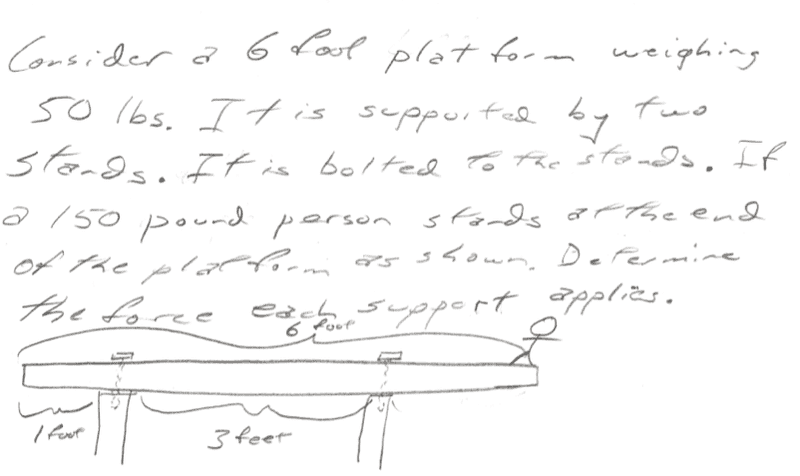# Finding forces on bolted platform (torques/forces)

## Homework StatementƩF = mA
T=rxF

## The Attempt at a Solution

Using sum of forces, I found:
ƩF=mA
150lb + 50lb - F1 - F2 = 0
(calling the support on the right "1") ƩT1 = 0

But I'm a little confused on where to go from this point. Any advice?

Look at each of the two supports individually. What forces act on each?

That would be the upward force of the board due to the torque at support 1, minus the force of the bolt holding the board to the support, correct?

And what can you say about the sum of the torques?

The sum of the torques, because there is no motion, will equal zero. Would it be correct to say the following...?
ƩT=0
0=(150lb)(2ft)-(50lb)(1ft)+(F2)(3ft)
F2=83.3lb